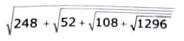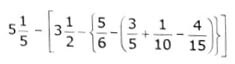# UTET Exam Nov 2021 Paper – 1 (Primary Level) | Part – IV – Mathematics

## Part – IV – Mathematics

91. If 2160 = 22a x 3b x 5c, then the value of 3a x 2-b x 5-c will be –

(A) 81/40

(B) 37/39

(C) 1/2

(D) 0

92. If third Friday is on 16th of month then what will be the date of fourth Tuesday of that month?

(A) 27

(B) 29

(C) 20

(D) 22

93. If the length and breadth of a room is decreased by 10% and 20% respectively, while height is increased by 5%, then what percentage will be changes in the volume of the room?

(A) 24.4%

(B) 24.6%

(C) 24%

(D) 24.2%

94. If number 5132416p is divisible by 3, then which digit will come in place of p?

(A) 8

(B) 6

(C) 7

(D) 9

95. The value ofwill be –

(A) 14

(B) 12

(C) 16

(D) 13

96. When the number 398 is divided by 5, then the remainder is –

(A) 1

(B) 2

(C) 3

(D) 4

97. In how many hours, the number of seconds is equal to the number of minutes in 10 days –

(A) 2 hours

(B) 3 hours

(C) 4 hours

(D) 5 hours

98. If

then the value of x and y are –

(A) x = 9; y = 3

(B) x = 3; y = 9

(C) x = 5; y = 8

(D) x = 4; y = 8

99. The square root of (7 + 2√6) is –

(A) √6 + 1

(B) √3 + 2

(c) √2 + √5

(D) 2 + √5

100. The volume of a cube is 64 cm. The total surface area of the cube will be

(A) 12 cm2

(8) 24 cm2

(C) 48 cm2

(D) 96 cm2

101. A right angled triangle cannot be –

(A) An equilateral triangle

(B) An isosceles triangle

(C) A scalene triangle

(D) A polygon

102. The greatest five digit perfect square number is –

(A) 99999

(B) 99976

(C) 99856

(D) 99764

103. The value ofwill be –

(A) 21/10

(B) 17/10

(C) 8/10

(D) 12/10

104. How many natural numbers lie between 3 and 200 which are divisible by 7?

(A) 2

(B) 28

(C) 29

(D) 30

105. If HCF (a, 8) = 4 and LCM (a, 8) = 24, then the value of ‘a’ is –

(A) 14

(B) 8

(C) 10

(D) 12

106. In a circular park of diameter 80m, there is a square shaped playground of maximum area. The area of the playground is –

(A) 6400 m2

(B) 3200 m2

(C) 1600 m2

(D) 12800 m2

107. The method in which new rules are established by examples is –

(A) Deductive Method

(B) Inductive Method

(C) Analytical Method

(D) Synthesis Method

108. The oldest branch of Mathematics is –

(A) Algebra

(B) Arithmetic

(C) Geometry

(D) Astrology Math

109. What is the importance of Mathematics at Primary level?

(A) Cultural

(B) Social

(C) Religious

(D) Mental

110. According to Hogben – “Mathematics is the mirror of Civilization.” This statement corresponds to which value of Mathematics?

(A) Cultural Value

(B) Social Value

(C) Disciplinary Value

(D) None of these

111. If x:y = 1:2, then the value of (8x + 9y) : (8x + 2y) will be –

(A) 22 : 29

(B) 29 : 22

(C) 13 : 6

(D) 6 : 13

112. If a metallic cone of radius 30 cm and height 45 cm is melted and recast into metallic spheres of radius 5 cm, find the number of spheres –

(A) 81

(B) 41

(C) 80

(D) 40

113. A work can be completed by P and Q in 12 days, Q and R in 15 days & R and P in 20 days. In how many days P alone can finish the work?

(A) 10

(B) 20

(C) 30

(D) 60

114. The average marks obtained by 40 students of a class is 86. If the 5 highest marks are removed, the average reduces by one mark. Find the average marks of top 5 students –

(A) 92

(B) 96

(C) 93

(D) 97

115. What is the major problem found in primary level teacher of mathematics?

(A) Lack of pedagogical training

(B) Lack of preparation

(C) Busyness in record maintenance

(D) None of these

116. Which of the following method is based on principles of “Watch, listen and learn”?

(A) Laboratory Method

(B) Demonstration Method

(C) Lecture Method

(D) Research Method

117. If x + y= 2a, then the value of a/(x – a) + a/(y – a) is –

(A) 2

(B) 2a2

(C) 0

(D) 2a

118. Zinc and Copper are in the ratio 5:3 in 400 gm. of an alloy. How much of copper (in gms) should be added to make the ratio 5 : 6?

(A) 50

(B) 150

(C) 550

(D) 300

119. If 1 is subtracted to both the numerator and the denominator of a fraction, it becomes 1/3. If 1 is added to both the numerator and denominator of that fraction, it becomes 1/2. Then the sum of numerator and denominator of that fraction is –

(A) 10

(B) 18

(C) 7

(D) 16

120. A car can finish a certain journey in 10 hours at speed of 48 km/h. In order to cover the same distance in 8 hours, the speed of the car must be increased by –

(A) 6 Km/h

(B) 12 km/h

(C) 15 Km/h

(D) 60 Km/h